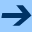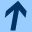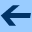The functional equation of the mean sun written as a group action

## The functional equation of the mean sun written as a group action

Grazer Mathematische Berichte 344, 25 - 30, 2001.

Abstract: A generalization g(s+t)x(u)=g(s)x(t+u) (for all s,t,u in R ) of the functional equation of the mean sun is studied, where a group G acts on a set X, (R,+) is a not necessarily commutative group and both x: R→ X and g: R→ G are unknown functions, which will be determined by the equation.

harald.fripertinger "at" uni-graz.at, October 10, 2019